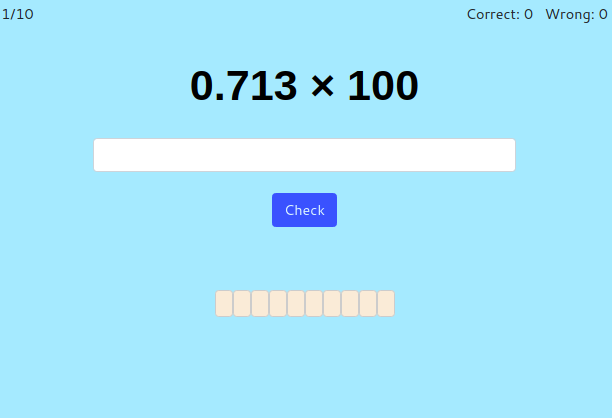MM Practice
×
Multiplication
Division
Place Value
Fractions & Decimals
Measurement
Statistics & Probability
Pre-Algebra
Money
Kindergarten
Geometry

# Multiply and Divide Decimals by powers of 10Online practice for grades 5-7

Here you can practice multiplying and dividing decimals by powers of ten (by numbers such as 10, 100, 1000, and so on).

The shortcut for doing this is to move the decimal point as many places as there are zeros in the power of ten. But this rule is actually based on place value. In reality, it's not the POINT that is moving, but the NUMBER itself is moving within the different places.

You can choose timed or untimed practice, the number of practice problems, the maximum number of decimal digits used, and the maximum power of ten.

10

Screenshot:OR
Practice for a set time: min

Allow my comment to be posted on this site